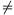Mathematical and Physical Journal
for High Schools
Issued by the MATFUND Foundation
 Already signed up? New to KöMaL?

# KöMaL Problems in Mathematics, May 2011

Show/hide problems of signs:## Problems with sign 'C'

Deadline expired on June 10, 2011.

C. 1080. A steerable airship has two engines and a given supply of fuel. If both engines are operated, the airship covers 88 kilometres per hour. If the first engine were used only, the fuel would last 25 hours longer, but the distance covered per hour would only be 45 km. If the second engine were used only, the fuel would last 16 hours longer than with two engines, and the distance covered per hour would only be 72 km. In which case can the airship travel the longest distance?

(5 pont)

solution (in Hungarian), statistics

C. 1081. Two regular polygons are said to be matching if the double of the interior angle of one of them equals the triple of the exterior angle of the other. Find all pairs of matching polygons.

(5 pont)

solution (in Hungarian), statistics

C. 1082. The first digit of a six-digit number is transferred to the end of the number. Then the first digit of the resulting six-digit number is, again, transferred to the end of the number. The six-digit number obtained in this way is three times the previous number andtimes the original number. What is the original six-digit number?

(5 pont)

solution (in Hungarian), statistics

C. 1083. The length of one side of a triangle is 8 cm, one of the angles lying on it is 60o, and the radius of the inscribed circle iscm. How long are the other two sides?

(5 pont)

solution (in Hungarian), statistics

C. 1084. The point P(8;4) divides a chord of the parabola y2=4x in a 1:4 ratio. Find the coordinates of the endpoints of the chord.

(5 pont)

solution (in Hungarian), statistics## Problems with sign 'B'

Deadline expired on June 10, 2011.

B. 4362. Each vertex of a solid cube is cut off. The faces of the resulting polyhedron are 8 triangles and 6 heptagons. What are the possible numbers of vertices and edges of such polyhedra?

(3 pont)

solution (in Hungarian), statistics

B. 4363. The reciprocals of the natural numbers 2 to 2011 are written on a blackboard. In each step, two numbers x and y are erased and replaced with the number. This step is repeated 2009 times, until a single number remains. What may the remaining number be?

(Suggested by B. Kovács, Szatmárnémeti)

(4 pont)

solution (in Hungarian), statistics

B. 4364. Let abc>0. Prove that.

(Suggested by J. Mészáros, Jóka)

(4 pont)

solution (in Hungarian), statistics

B. 4365. Find all positive integers n such that 2n-1 and 2n+2-1 are both primes, and 2n+1-1 is not divisible by 7.

(Suggested by S. Kiss, Budapest)

(3 pont)

solution (in Hungarian), statistics

B. 4366. Let M denote the orthocentre of the acute-angled triangle ABC, and let A1, B1, C1, respectively, denote the circumcentres of triangles BCM, CAM, ABM. Prove that the lines AA1, BB1 és CC1 are concurrent.

(4 pont)

solution (in Hungarian), statistics

B. 4367. Solve the following equation:.

(Suggested by J. Mészáros, Jóka)

(4 pont)

solution (in Hungarian), statistics

B. 4368. Let D, E and F, respectively, be points on sides AB, BC, CA of a triangle ABC such that AD:DB=BE:EC=CF:FA1. The lines AE, BF, CD intersect one another at points G, H, I, respectively. Prove that the centroids of triangles ABC and GHI coincide.

(Suggested by Sz. Miklós, Herceghalom)

(3 pont)

solution (in Hungarian), statistics

B. 4369. Each of the circles k1, k2 and k3 passes through a point P, and the circles ki and kj also pass through the point Mi,j. Let A be an arbitrary point of circle k1. Let k4 be an arbitrary circle passing through A and M1,2, and let k5 be an arbitrary circle passing through A and M1,3. Show that if the other intersections of the pairs of circles k4 and k2, k5 and k3, k4 and k5 are B, C and D, respectively, then the points M2,3, B, C, D are either concyclic or collinear.

(4 pont)

solution (in Hungarian), statistics

B. 4370. Let a, b, c denote the lengths of the sides of a triangle, and let u, v, w, respectively, be the distances of the centre of the incircle from the vertices opposite to the sides. Prove that.

(Suggested by J. Mészáros, Jóka)

(5 pont)

solution (in Hungarian), statistics

B. 4371. Prove that(Suggested by B. Kovács, Szatmárnémeti)

(5 pont)

solution (in Hungarian), statistics## Problems with sign 'A'

Deadline expired on June 10, 2011.

A. 536. The positive real numbers a, b, c, d satisfy a+b+c+d=abc+abd+acd+bcd. Prove that(5 pont)

statistics

A. 537. The edges of the complete graph on n vertices are labeled by the numbersin such a way that each number is used exactly once. Prove that if n is sufficiently large then there exists a (possible cyclic) path of three edges such that the sum of the numbers assigned to these edges is at most 3n-1000.

(Kolmogorov Cup, 2009; a problem by I. Bogdanov, G. Chelnokov and K. Knop)

(5 pont)

statistics

A. 538. In the 3-dimensional hyperbolic space there are given a planeand four distinct lines a1, a2, r1, r2 in such positions that a1 and a2 are perpendicular to, r1 is coplanar with a1, r2 is coplanar with a2, finally r1 and r2 intersectat the same angle. Rotate r1 around a1 and rotate r2 around a2; denote byandthe two surfaces of revolution they sweep out. Show that the common points ofandlie in a plane.

(5 pont)

statistics